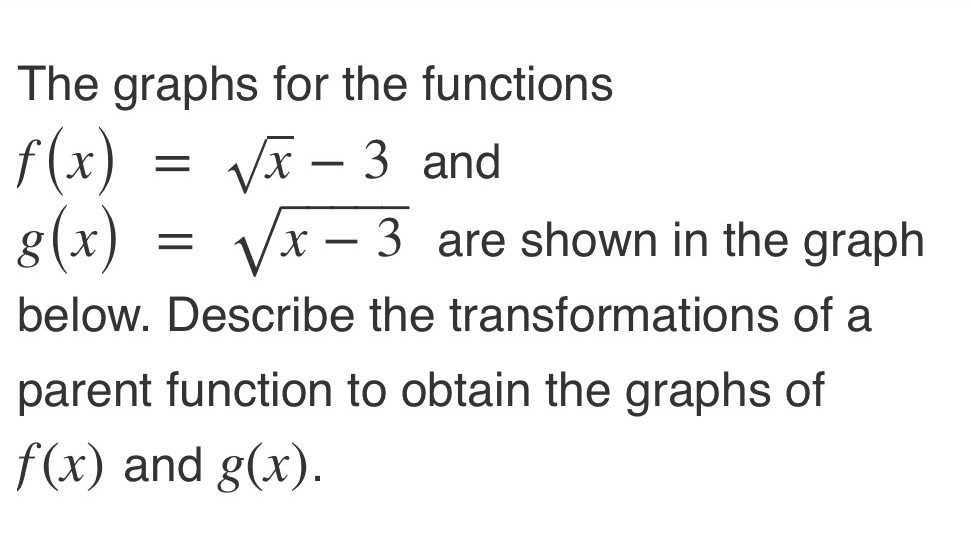### Still have math questions?

Algebra
QuestionThe graphs for the functions $$f ( x ) = \sqrt { x } - 3$$ and $$g ( x ) = \sqrt { x - 3 }$$ are shown in the graph below.

Describe the transformations of a parent function to obtain the graphs of

$$f ( x )$$ and $$g ( x )$$ .